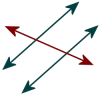Games
Problems
Go Pro!

Lesson Plans > Mathematics > Geometry > LogicOne thing that many geometry textbooks don't adequately address is the question of what kinds of assumptions we can make based on a diagram that is given to us in a problem. Most books will emphasize that diagrams are not drawn to scale, and students may be left thinking that you can't assume anything based on a diagram. However, this is not the case. So we spend some time early on discussing the do's and don'ts of diagram interpretation.

Assumptions you can't make

• If two segments look congruent, you may not assume that they are.
• If two segments don't look congruent, you may not assume that they aren't.
• If one segment looks longer than another, you may not assume that it really is.
• If an angle looks like a right angle, you may not assume that it is.
• If an angle looks acute or obtuse, you may not assume that it is.
• If two angles look congruent, you may not assume that they are.
• If two angles don't look congruent, you may not ssume that they aren't.
• If two lines appear to be parallel, you may not assume that they are.
• If two lines appear to not be parallel, you cannot assume that they aren't, unless their intersection point is part of the diagram.

The list below is things you can assume from a diagram. This list might not be universal; study your geometry text and see if this list matches the assumptions made based on diagrams in your book.

Assumptions you can make

• If three or more points appear to be collinear (they are connected by what appears to be a straight line), you may assume that they are collinear.
• If three points are collinear, the point which appears to be between the othe two really is between them.
• If a point appears to be on the interior of an angle, it really is on the interior.
• If a point appears to be on the exterior of an angle, it really is on the exterior.
Lesson by Mr. Twitchell

# Blogs on This SiteReviews and book lists - books we love!The site administrator fields questions from visitors.Like us on Facebook to get updates about new resources